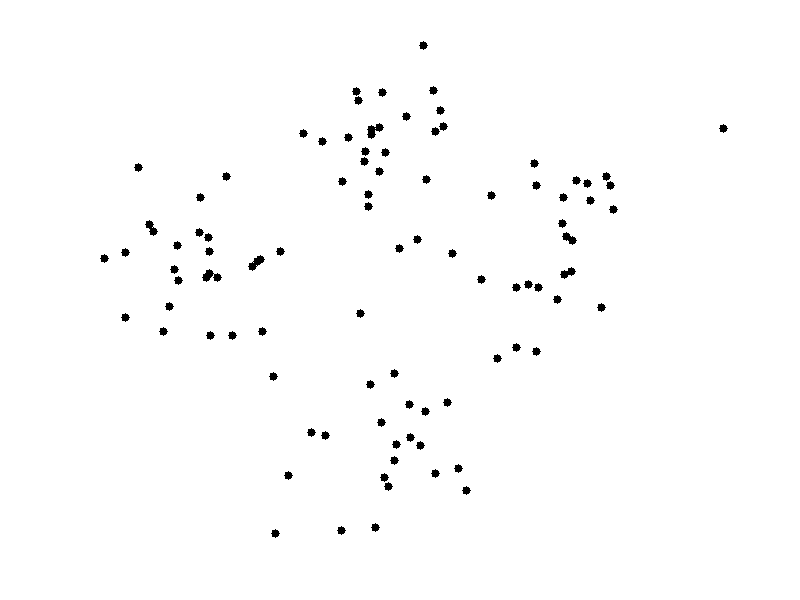# Anomaly detection and a simple algorithm with probabilistic approach.

What is anomaly detection? It is the way of detecting a outlier data point among the other points that have a some kind of logical distribution.  Outlier one is also anomalous point (Figure 1)Figure 1

What are the applications?

•  Fraud user activity detection - it is a way of detecting hacker activities on web applications or network connections by considering varying attributes of the present status. For example , an application can keep track of the user's inputs to website and the work load that he proposes to system. Considering these current attribute values detection system decide a particular fraud action and kick out the user if there is.
• Data center monitoring - You might be governing a data center with vast amount of computers so it is really hard to check each computer regularly for any flaw. A anomaly detection system might be working by considering network connection parameters of the computers, CPU and Memory Loads, it detect any problem on computer. Continue reading Anomaly detection and a simple algorithm with probabilistic approach.

# How K-means clustering works

K-means is the most primitive and easy to use clustering algorithm (also a Machine Learning algorithm).
There are 4 basic steps of K-means:

1. Choose K different initial data points on instance space (as initial centroids) - centroid is the mean points of the clusters that overview the attributes of the classes-.
2. Assign each object to the nearest centroid.
3. After all the object are assigned, recalculate the centroids by taking the averages of the current classes (clusters)
4. Do 2-3 until centroid are stabilized.

Caveats for K-means:

• Although it can be proved that the procedure will always terminate, the k-means algorithm does not necessarily find the most optimal configuration, corresponding to the global objective function minimum.
• The algorithm is also significantly sensitive to the initial randomly selected cluster centres. The k-means algorithm can be run multiple times to reduce this effect.

Here is the basic animation to show the intuition of K-means.# What are the needs of Machine Learning?

-Convexity, including convex optimization and formulation of problems as convex programs. Two important subsets of this are linear programming and proximal gradient-style optimization algorithms and formulations, which have a ridiculously vast array of applications for industrial engineering and machine learning.
-Probabilistic modeling and inference: Graphical models and max-entropy models are the most important, and have a vast array of applications in machine learning and more structured statistical modeling. Markov Chain Monte Carlo is a terrific and amazing algorithm with a great special case called Gibbs sampling - they both present almost generic methods of Continue reading What are the needs of Machine Learning?

# Other algorithms for optimization cost func. in Machine Learning

The most basic algorithm is Gradient Descent to optimize your cost function in a machine learning algorithm but it has some problems like;

• Choosing alpha value for the steps of convergence process.
• Too much iteration for converge.

There are some other approaches that are;

• Conjugate Gradient
• BFGS
• L-BFGS

# Machine Learning Terms (#3)

As a headline note, I am not writing these staff to give all the details and the information about the headings, also I am not qualified as this much. I just trying to underline some basic facts for the ones who are interested in machine learning and searching some facts to investigate. Thus my headings just small introduction for your ML world search.

Linear Regression: a basic algorithm to estimate continuous output value by considering the attributes of the given instance according to the given instances in data-set with their attribut Continue reading Machine Learning Terms (#3)

# Some Basic Machine Learning Terms #1If you are working on some project related to machine learning (ML) or you are a newbie researcher knowing these terms and definitions might be useful.

Machine Learning: A computer program is said to learn from experience E with respect to some class of tasks T and performance measure P, if its performance at tasks in T, as measured by P, improves with experience E -- Tom M. Mitchell Continue reading Some Basic Machine Learning Terms #1